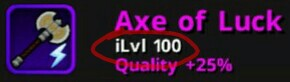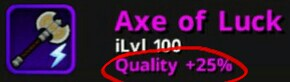# Formulas

what is the [1+item level] on BWD formula?This is the item level.This is item quality.so if the item level is 100 and the formula is 1+item level on bwd then (1+item level=101)?

Where did you get that formula?

This is the formula from codex.yeah, bwd*(1+item level*0.25) so how do you add 1+100 item level?
item level rather.

what

E=MC^2…

4 Likes

Lol

Lmao.

But what if Ensteins last words were: sorry it’s actually E=MC^3 in German.

2 Likes

Then all the Light Cycles from Tron would have engine trouble…?

2 Likes

This formula wrecks Energy units D:

2 Likes

Yep. Not to mention how out of whack the universe could be if that were the case of E=MC^3 instead of E=MC^2. It just couldn’t work. We’ll never knew what his actual last words were though in his death.

Although it would be fun imagining such an alternative universe , if it could even exist at all xD.

1 Like